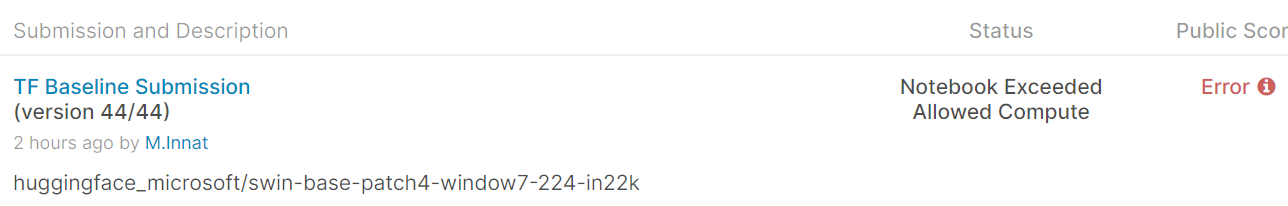# Potential memory leakage of TensorFlow Swin model on kaggle!

### System Info

Info:

``````Framework: TensorFlow 2 (Keras)
Version: 2.6
OS: Kaggle
``````

### Who can help?

Swin Model Card @amyeroberts
TensorFlow: @Rocketknight1
Vision: @NielsRogge, @sgugger

### Reproduction

A recent kaggle competition (hosted by Google), I tried to use pretrained `tf` swin transformer model from hugging face but even with the base model, I consistently received out of memory error. Below is the submission status with a `base_tf_swin` model.Some note:

• Other framework like pytorch works fine here.
• Other than this model, much larger model like `tf_convnext_xlarge` is able to run without OOM.

So, I’m assuming there might be some potential memory leakage in `tf_swin` implementation. Below is the code I use to build the complete model.

```id = "microsoft/swin-base-patch4-window7-224-in22k"

from transformers import AutoFeatureExtractor, TFSwinModel
feature_extractor = AutoFeatureExtractor.from_pretrained(id)```
```inputs = keras.Input(shape=(None, None, 3), dtype='uint8')
mode_inputs = tf.cast(inputs, tf.float32)

mode_inputs = keras.layers.Resizing(*INPUT_SHAPE)(mode_inputs)
mode_inputs = keras.layers.Rescaling(scale=1.0 / 255)(mode_inputs)
mode_inputs = keras.layers.Normalization(
mean=feature_extractor.image_mean,
variance=[x ** 2 for x in feature_extractor.image_std ],
axis=3
)(mode_inputs)
mode_inputs = keras.layers.Permute(dims=(3, 1, 2))(mode_inputs)

tf_huggingface_module = TFSwinModel.from_pretrained(id)
tf_huggingface_module.trainable = False```
```logits = tf_huggingface_module(mode_inputs)